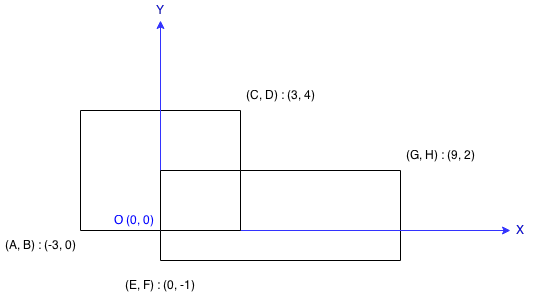07/16/2016 Math

## Question

Find the total area covered by two rectilinear rectangles in a 2D plane.

Each rectangle is defined by its bottom left corner and top right corner as shown in the figure.Assume that the total area is never beyond the maximum possible value of int.

## Solution

Result: Accepted Time: 20 ms

Here should be some explanations.

int max(const int a,const int b)
{
return a>b?a:b;
}
int min(const int a,const int b)
{
return a>b?b:a;
}
int computeArea(int A, int B, int C, int D, int E, int F, int G, int H) {

int sa = (C - A) * (D - B);
int sb = (G - E) * (H - F);
if( C < E || D < F || G < A || H < B)
return sa + sb;
int lx = max(A,E);
int ly = max(B,F);
int rx = min(C,G);
int ry = min(D,H);
int sc = (rx - lx) * (ry - ly);
return sa + sb - sc;
}


Complexity Analytics

• Time Complexity: $O(1)$
• Space Complexity: $O(1)$